# vs.eyeandcontacts.com

## Chapter 14  Symmetry Exercise 14.1

Question 1: Copy the figures with punched holes and find the axes of symmetry for the following:
(a)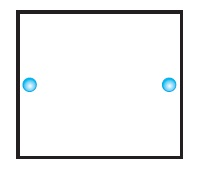(b)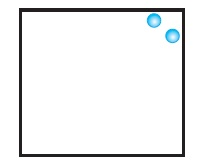(c)(d)(e)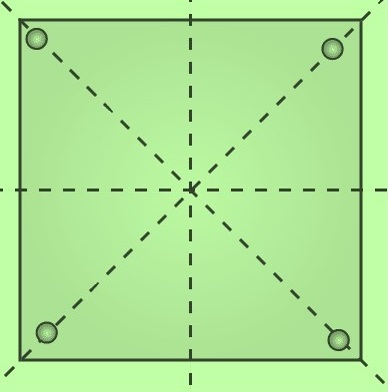(f)(g)(h)(i)(j)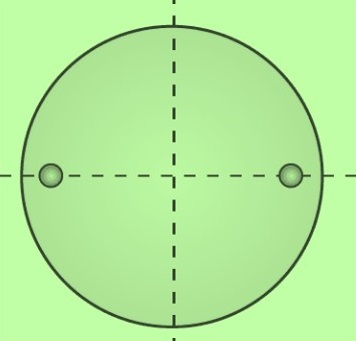(k)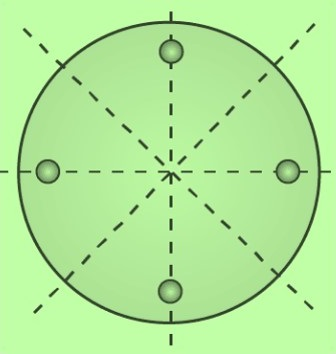(l)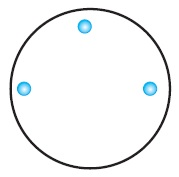Question 2: Given the line(s) of symmetry, find the other hole(s):
(a)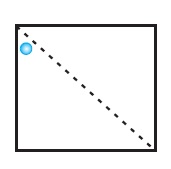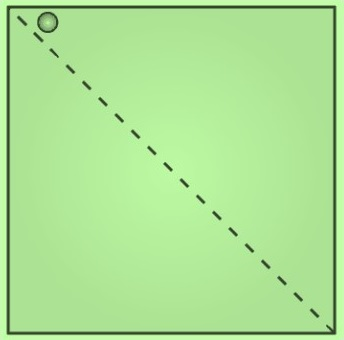(b)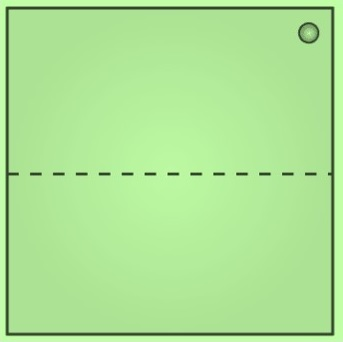(c)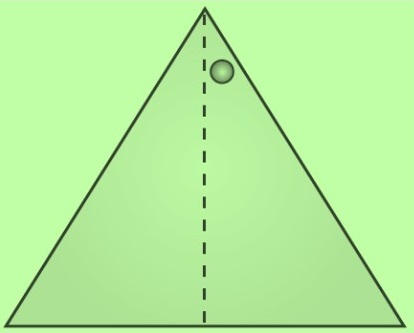(d)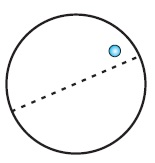(e)Question 3: In the following figures, the mirror line (i.e., the line of symmetry) is given as a dotted line. Complete each figure performing reflection in the dotted (mirror) line. (You might perhaps place a mirror along the dotted line and look into the mirror for the image). Are you able to recall the name of the figure you complete?
(a)Name of the figure is square.

(b)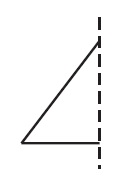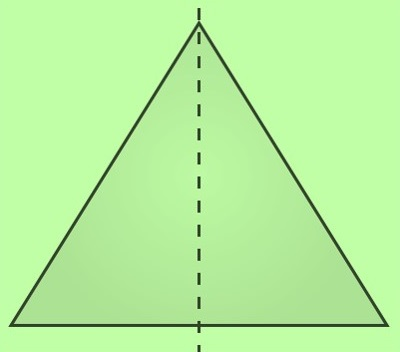Name of the figure is triangle.

(c)Name of the figure is rhombus.

(d)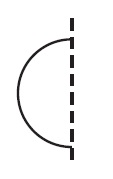Name of the figure is circle.

(e)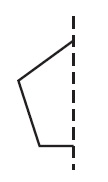Name of the figure is pentagon.

(f)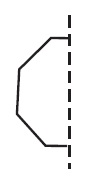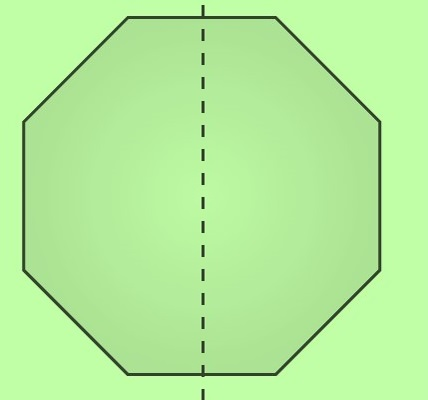Name of the figure is octagon.

Question 4: The following figures have more than one line of symmetry. Such figures are said to have multiple lines of symmetry.Identify multiple lines of symmetry, if any, in each of the following figures:
(a)Answer: The figure given has 3 lines of symmetry. So, it has multiple lines of symmetry.

(b)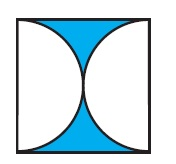The figure given has 2 lines of symmetry. So, it has multiple lines of symmetry.

(c)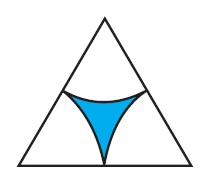The figure given has 3 lines of symmetry. So, it has multiple lines of symmetry.

(d)The figure given has 2 lines of symmetry. So, it has multiple lines of symmetry.

(e)The figure given has 4 lines of symmetry. So, it has multiple lines of symmetry.

(f)The figure given has only 1 line of symmetry.

(g)The figure given has 4 lines of symmetry. So, it has multiple lines of symmetry.

(h)The figure given has 6 lines of symmetry. So, it has multiple lines of symmetry.

Question 5: Copy the figure given here.
Take any one diagonal as a line of symmetry and shade a few more squares to make the figure symmetric about a diagonal. Is there more than one way to do that? Will the figure be symmetric about both the diagonals?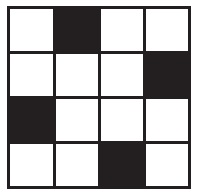Yes, the figure will be symmetrical about both diagonals.Yes, the figure can be made symmetrical by more than one way.

Question 6: Copy the diagram and complete each shape to be symmetric about the mirror line(s):
(a)(b)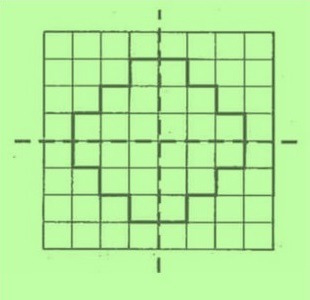(c)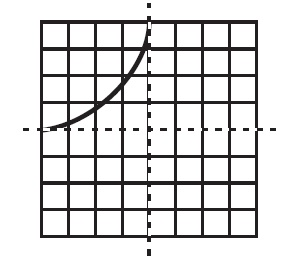(d)Question 7: State the number of lines of symmetry for the following figures:
(a) An equilateral triangle
An equilateral triangle has 3 lines of symmetry.

(b) An isosceles triangle
An isosceles triangle has 1 lines of symmetry.

(c) A scalene triangle
A scalene triangle has no line of symmetry.

(d) A square
A square has 4 lines of symmetry.

(e) A rectangle
A rectangle has 2 lines of symmetry.

(f) A rhombus
A rhombus has 2 lines of symmetry.

(g) A parallelogram
A parallelogram has no line of symmetry.

A quadrilateral has no line of symmetry.

(i) A regular hexagon
A regular hexagon has 6 lines of symmetry.

(j) A circle
A circle has infinite lines of symmetry.

Question 8: What letters of the English alphabet have reflectional symmetry (i.e., symmetry related to mirror reflection) about.
(a) A vertical mirror
(b) A horizontal mirror
(c) Both horizontal and vertical mirrors
a) A vertical mirror - A, H, I, M, O, T, U, V, W, X, Y
b) A horizontal mirror - B, C, D, E, H, I, K, O, X
c) Both horizontal and vertical mirrors - H, I, O, X

Question 9: Give three examples of shapes with no line of symmetry.
A scalene triangle, a quadrilateral and a parallelogram

Question 10: What other name can you give to the line of symmetry of
(a) An isosceles triangle?
The other name to the line of symmetry of an isosceles triangle is median or an altitude.

(b) A circle?